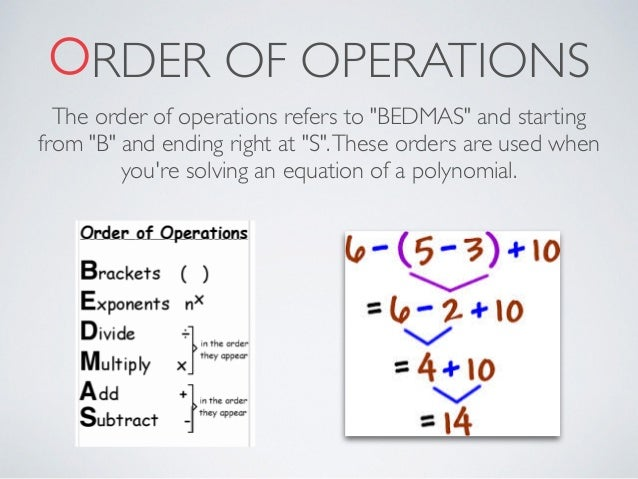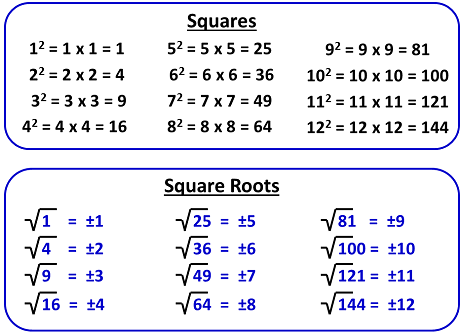# Maths 8Practice Test – Mathematics for Australia 8

3 proportional

3.1using ration to divide Quantities

 Week Term 1 Term 2 Term 3 Term 4 1 Number Equations The Geometry of Polygons Formative and Summative Topic Test 2- Coordinate Geometry/ Algebra: Patterns and Formulae/Problem Solving. 2 Number / Real Numbers Equations   Year 8 Camp The Geometry of Polygons/ Measurement: Length and Area Probability 3 Real Numbers Formative and Summative Topic Test 2- Algebraic Operations, Laws of Algebra, Equations. Year 8 Camp Measurement: Length and Area Probability/Statistics 4 Sets and venn Diagrams Percentage    Book Mark 2 Further Measurement Statistics Book Mark 2 5 Sets and venn Diagrams   Book Mark 1 Percentage/ Ratio and Proportion Further Measurement   Book Mark 1 Formative and Summative Topic Test 3- Probability/Statistics 6 Formative and Summative Topic Test 1- Number, Real Numbers, Sets and venn Diagrams Ratio and Proportion Formative and Summative Topic Test 1-The Geometry of Polygons/ Measurement: Length and Area/ Further Measurement Congruences and Transformations 7 Algebraic Operations Formative and Summative Topic Test 3- Percentage/ Ratio and Proportion Coordinate Geometry Revision 8 Algebraic Operations/ Laws of Algebra Rates Coordinate Geometry/ Algebra: Patterns and Formulae Revision Semester Examination 9 Laws of Algebra Revision Algebra: Patterns and Formulae 10 Revision Semester Examination Problem Solving# HCFSQUARES AND